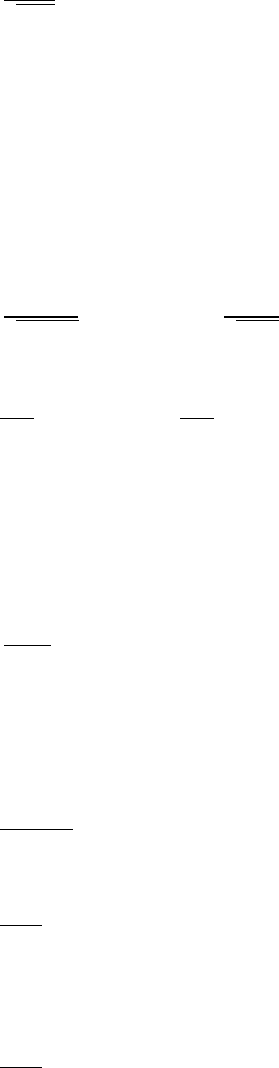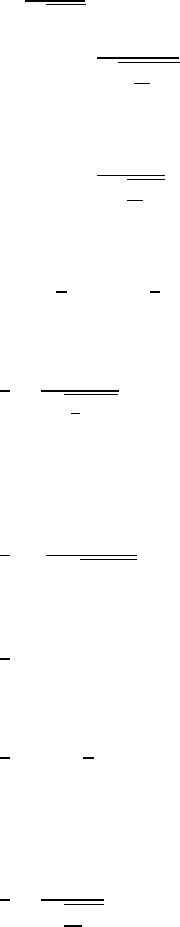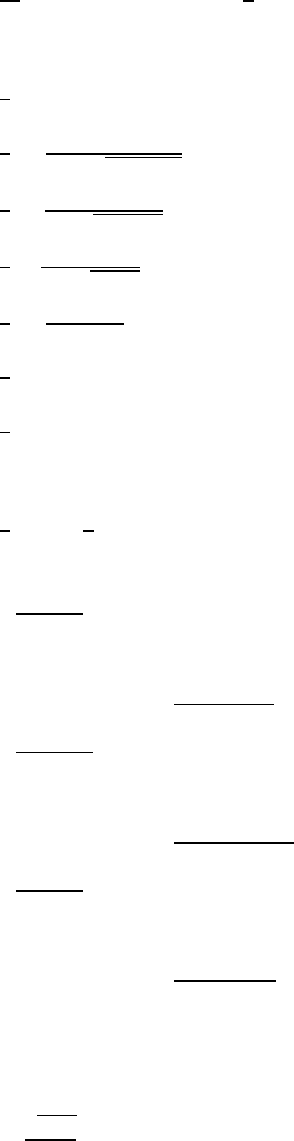Study Guides (390,000)
US (220,000)
UC-Irvine (3,000)
MATH (600)
MATH 2B (100)
All (60)
Midterm

# MATH 2B Study Guide - Midterm Guide: Pythagorean TheoremPremium

Department
Mathematics
Course Code
MATH 2B
Professor
All
Study Guide
Midterm

This preview shows pages 1-2. to view the full 8 pages of the document.Section 7.3 - Trig Substitution
Example #1
How do we integrate well-known trig functions the easy way?
1
12
= () +
Can we do this in a much more difficult way? Yes!
Use substitution of a different kind
= , = 
Set the substitution variables
=    () =
1
12 =
1
2
Substitute and simplify
1
 =


 = +
() +
Remember to replug () for
Example #2
1
1 + 2, we know from memorization is () + , but can we make this more
difficult? Yes
= , = 
Make the substitution and derivative
1
1 + 2(2)
Plug in the substitution
1
2(2)
Use trig identity
1 +  = 
2
2

Only pages 1-2 are available for preview. Some parts have been intentionally blurred.

Subscribers OnlySimplify
 = +
Take the integral
() +
Remember to replug in
Example #3
1
24
1
4(2
41) 
We can rewrite the bottom half
1
22
41
Pull the 4 out of the square root, changing it to 2
=
, =
, 2 = 
Set your substitutions, and rearrange them for usage
(
)
Then we can simplify further and pull out the constants from the integral
itself
()2
Set the know substitutions
() +
Take the integral
(
) +
Plug the information back into the substitutions
Example #4


Unlock to view full version

Subscribers Only

Only pages 1-2 are available for preview. Some parts have been intentionally blurred.

Subscribers OnlyFrom here, there’s another way to solve this problem
= ,
= 
Set substitutions
 = 
  (2)







+
Replug in substitutions
(
) +
Rules
1
If there exists a part of the integral in this format, let = 
Because 1 = 
1 +
If there exists a part of the integral in this format, let = 
Because 1 +  =
1
If there exists a part of the integral in this format, let = 
Because 1 = 
Example #5
12
2
Set up the substitutions